# Excel Case: Poke Mart Shop Cart

## Case: Poke Mart Shop Cart

In this case we help the Poke Mart merchant to create a shop cart overview with prices, discount and total price. You will practice the skills that you have learned in the earlier chapters.

Copy values or type the following data: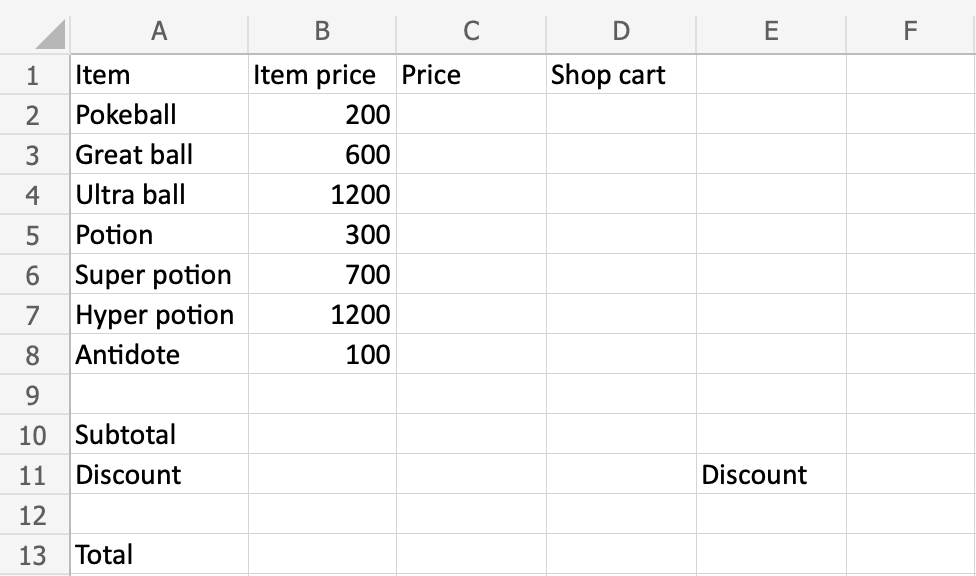Copy Values

To solve the case we need to complete the following:

• Prices for items (`C2:C8`)
• Sub total (`B10`)
• Add discount (`B11`)
• Total price (`B13`)

Start by creating the first formula for `Item price*Shop` cart. We want the price to change when we change items in the Shop cart. Use the fill function on the range `D2:D8` afterwards to save time.

Step by step:

1. Select `C2`, type (`=`)
2. Select `B2`
3. Type (`*`)
4. Select `D2`
5. Hit enter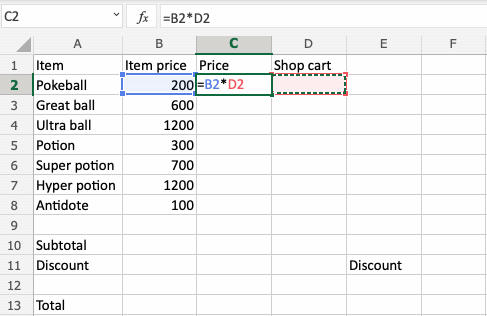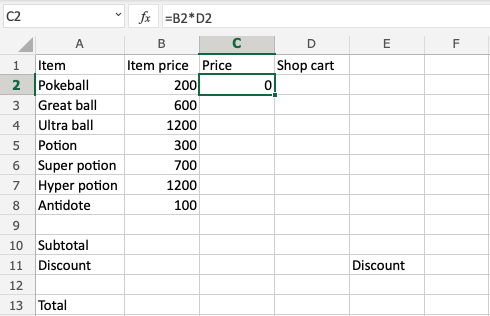Test the formula by Typing `D2(1)`: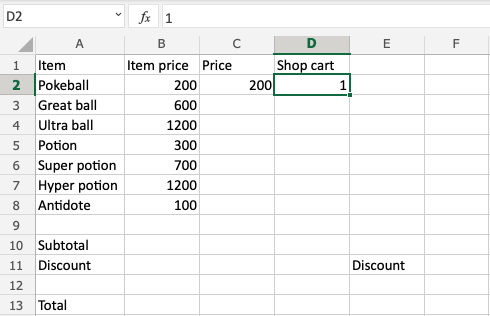Did you get `C2(200)`? That matches the price for one Pokeball! Good job!

We need the same formula for `C2:C8`, let's fill it downwards!

Note: We need relative references as we want the fill function to continue the formula to the next rows.Fill `D2:D8` to double check the prices:That looks right!

Let us find the subtotal by using the `SUM` function on the `C2:C8` range.

1. Type `B10(=SUM)`
2. Double click the `SUM` function
3. Mark the range `C2:C8`
4. Hit enter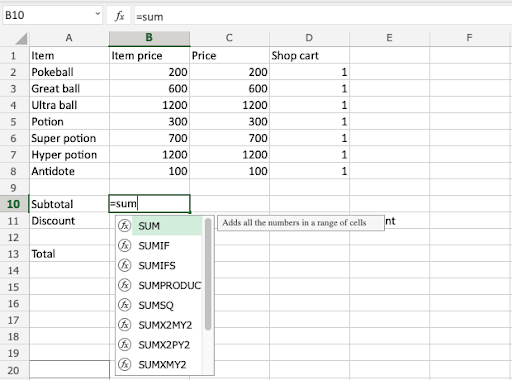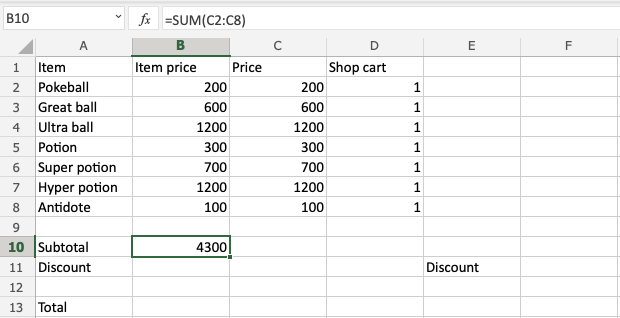Well done! You have successfully calculated the subtotal using the `SUM` function.

Next, let's add a `10%` discount. Type `F11(10)`:Apply the discount to the subtotal:

1. Select `B11`, type (`=`)
2. Type `(F11/100)*B10`
3. Hit enter

Note: The `(F11/100)` was used to change the value `10` to `0.1`, which is `10%`.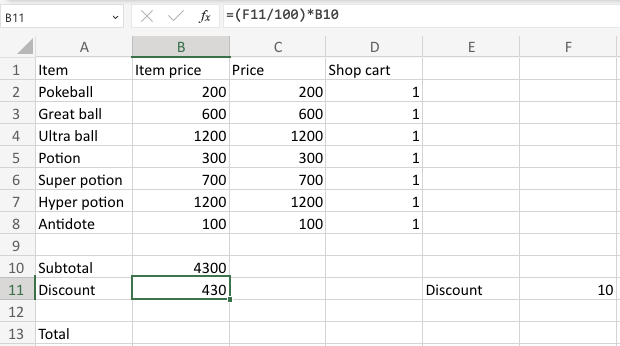Subtract the discount `B11` from the subtotal `B10` to calculate the total.

1. Select B13 type (`=`)
2. Select the minuend `B10`
3. Type (`-`)
4. Select the subtrahend `B11`
5. Hit enter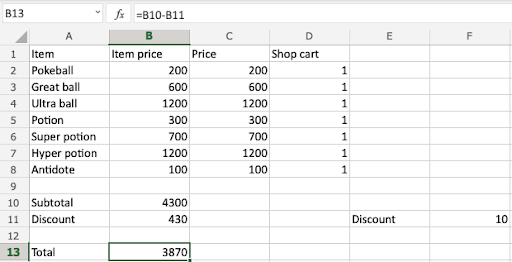That is great!

You have completed all the tasks for the Poke Mart merchant.

• Prices for items
Range `C2:D8` calculates the prices by multiplying item price and shop cart

• Sub total
The `SUM` function adds the range `C2:C8`

The discount is calculated by using `F11(10)` into the formula in `B11`

• Total price
Total price is calculated by subtracting the discount from the subtotal

Try changing the amounts in the shop cart and discount to see how the calculations adapt!Case completed! Good job!

W3Schools is optimized for learning and training. Examples might be simplified to improve reading and learning. Tutorials, references, and examples are constantly reviewed to avoid errors, but we cannot warrant full correctness of all content. While using W3Schools, you agree to have read and accepted our terms of use, cookie and privacy policy.